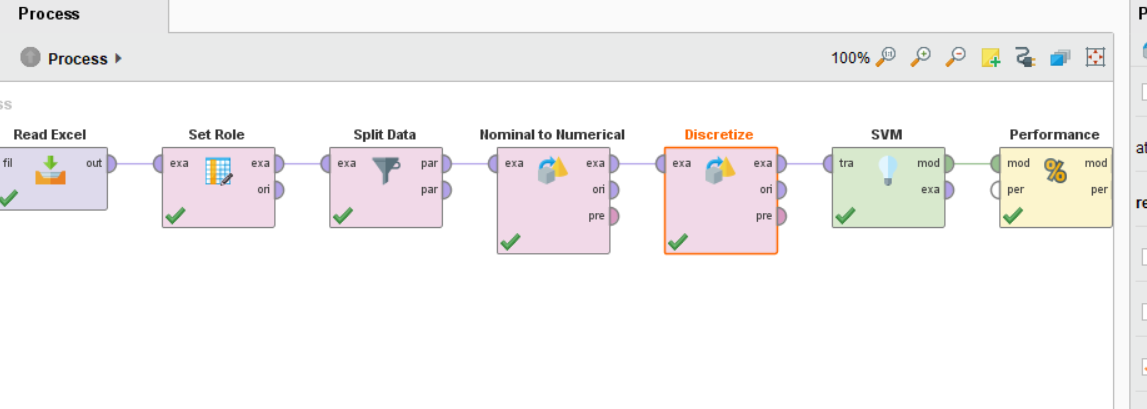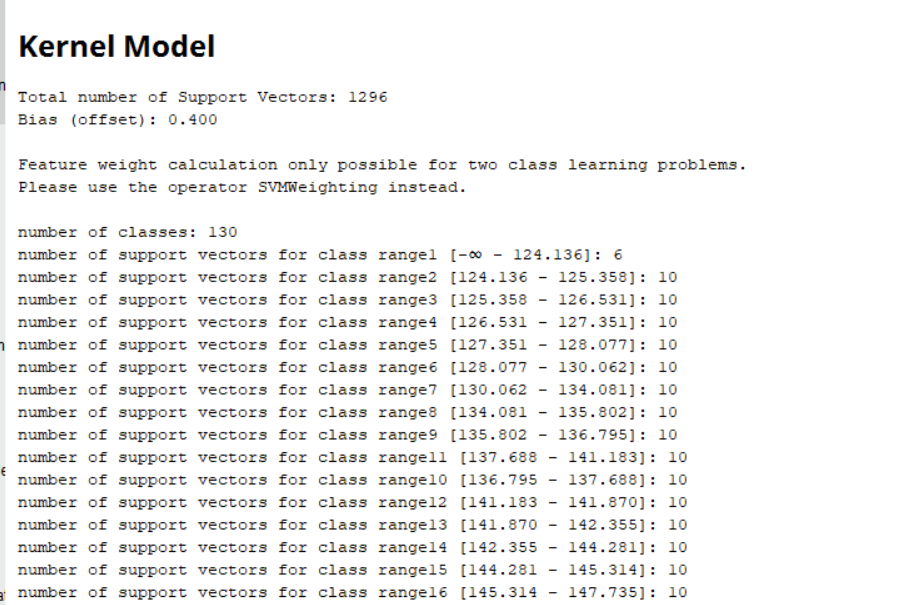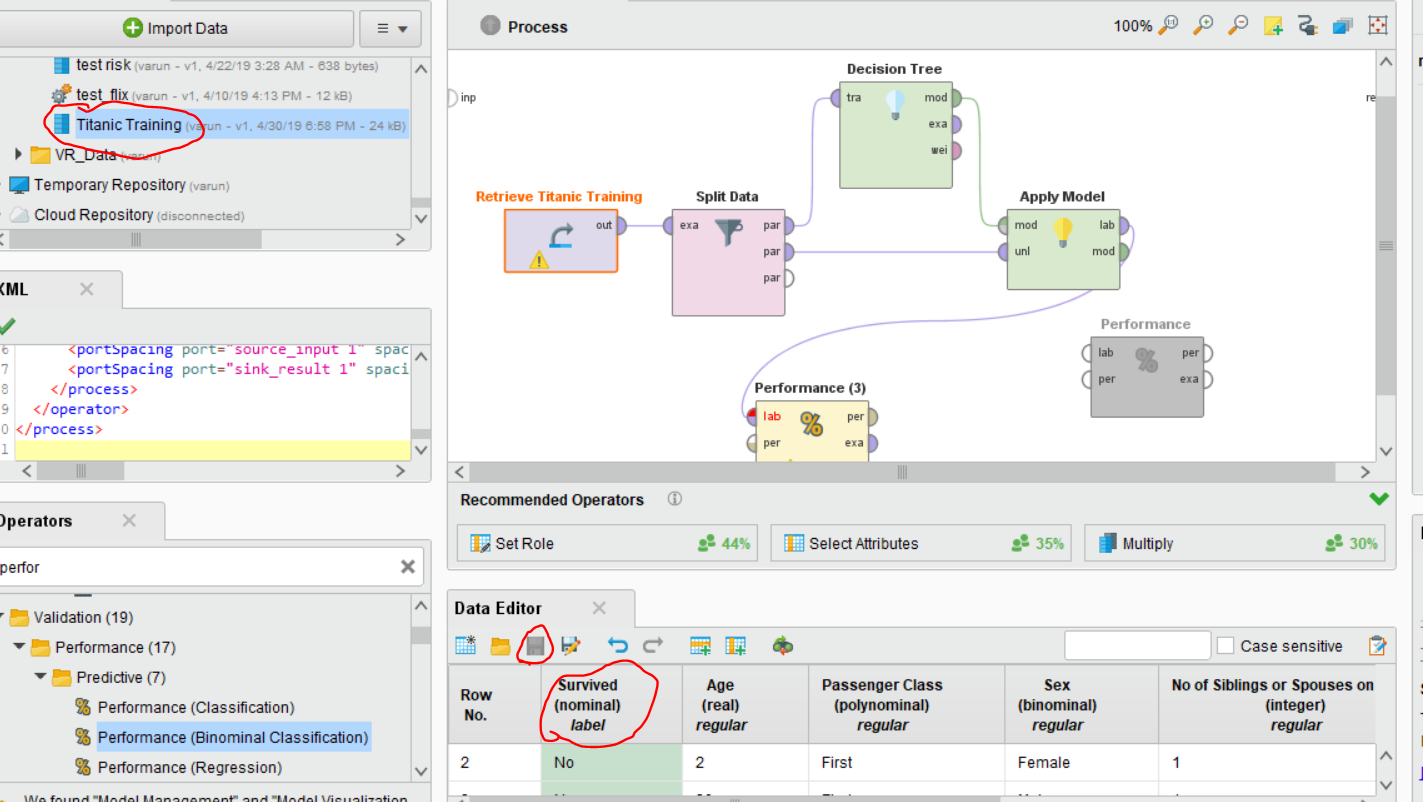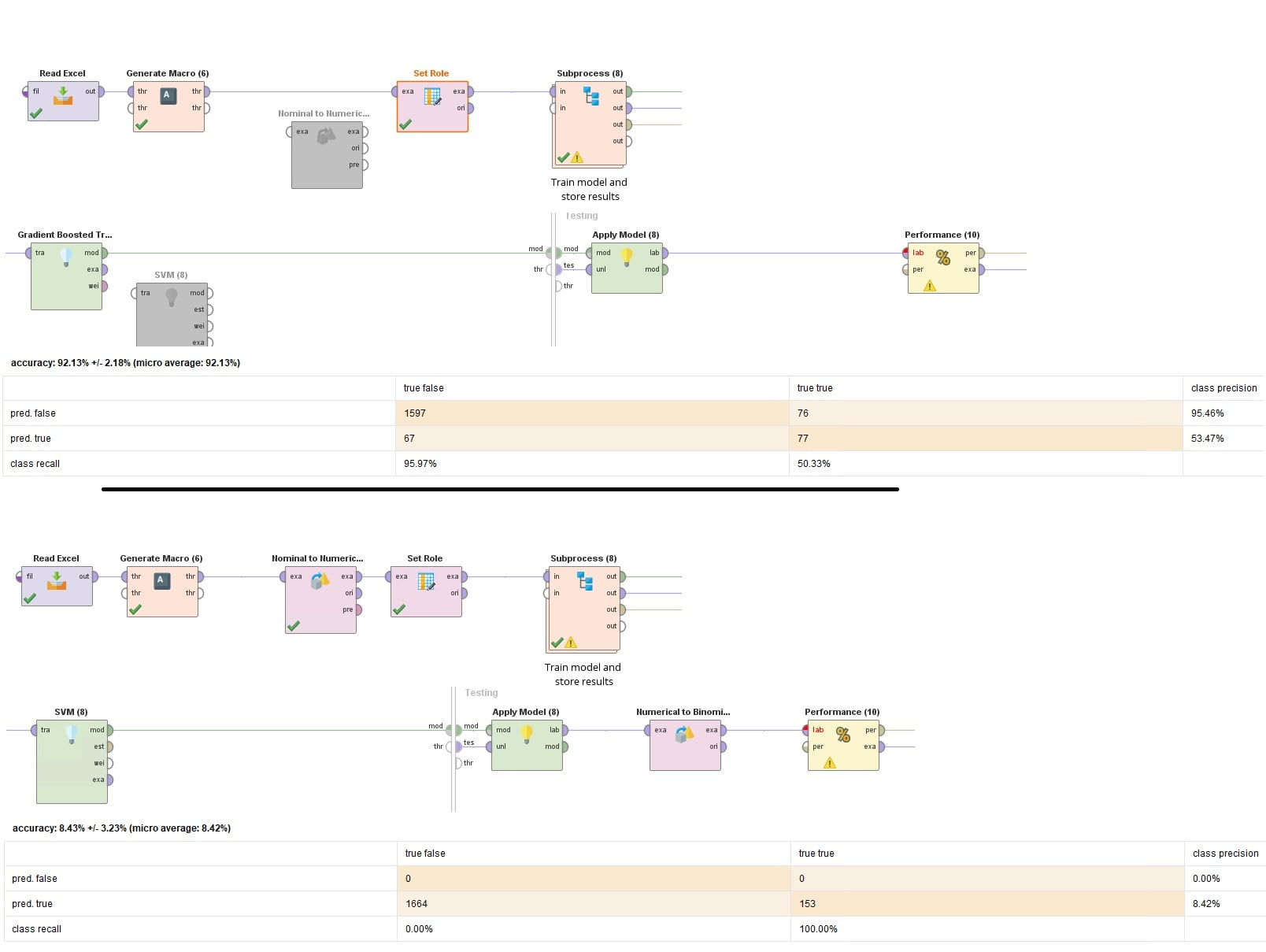# svm

Posts: 0Learner III
Hi
how can we use svm in polynominal label ?

• Administrator, Moderator, Employee, RapidMiner Certified Analyst, RapidMiner Certified Expert, University Professor Posts: 3,120RM Data Scientist
Solution Accepted
Hi,
i would recommend to use the operator Polynominal by Binominal Classification for this.

Best,
Martin
- Head of Data Science Services at RapidMiner -
Dortmund, Germany
• Moderator, Member Posts: 1,207Unicorn
edited April 2019 Solution Accepted
There are two options, you can either classify with a variant of SVM operator called LibSVM (you can find in operators) that can handle multiple classes or use a polynomial to the binomial operator that divides classes and do one on all classification.

You can take a look at the operators and in help, you can find a tutorial which shows how you can apply these operators.

Thanks
Regards,
Varun
https://www.varunmandalapu.com/

# Be Safe. Follow precautions and Maintain Social Distancing

• Moderator, Member Posts: 1,207Unicorn
Solution Accepted
@mbs

Provide @Noel with your XML code if possible. You can copy and paste that from View --> Show Panel --> XML or export your process and attach it here so that he can import. This would help more compared to screenshotsRegards,
Varun
https://www.varunmandalapu.com/

# Be Safe. Follow precautions and Maintain Social Distancing

• Moderator, Member Posts: 1,207Unicorn
Solution Accepted
Regards,
Varun
https://www.varunmandalapu.com/

# Be Safe. Follow precautions and Maintain Social Distancing

• Moderator, Member Posts: 1,207Unicorn
SVM(libSVM) does work on polynomial labels
Regards,
Varun
https://www.varunmandalapu.com/

# Be Safe. Follow precautions and Maintain Social Distancing

• Posts: 0Learner III
plz explain it moreThank you
• Posts: 0Learner III
edited April 2019
Thank you every body.
I will try all of your suggestions.• Member Posts: 82Maven
Hi Martin-

I found myself in a similar situation and, as you suggested, I used the Nominal to Binominal operator. I still get an error, though, "Insufficient capability... SVM does not have sufficient capabilities for the given data set: binominal attributes not supported".

Any thots (process and data attached, if helpful)?

Thanks,
Noel
• Posts: 0Learner III
edited April 2019
Hi @ Noel
• Posts: 0Learner III
edited April 2019
this is the solution with your excel• Posts: 0Learner III
And this is one example with your data• Member Posts: 82Maven
@mbs- Thanks for the replies. Much appreciated! Hopefully I’ll be able to replicate your solution later this afternoon.
• Member Posts: 82Maven
@mbs- Thanks again. Unfortunately, I'm a newbie and am still having some trouble. Which flavors of Discretize and Performance did you use? What parameters did you use for the Nominal to Numerical and Discretize? (Would you be opposed to posting the process?)
• Posts: 0Learner III
@varunm1
sure I will do it• Posts: 0Learner III
edited April 2019
@Noel
sorry because of delay. please look at the XML<?xml version="1.0" encoding="UTF-8"?><process version="9.2.001">
<context>
<input/>
<output/>
<macros/>
</context>
<operator activated="true" class="process" compatibility="9.2.001" expanded="true" name="Process">
<parameter key="logverbosity" value="init"/>
<parameter key="random_seed" value="2001"/>
<parameter key="send_mail" value="never"/>
<parameter key="process_duration_for_mail" value="30"/>
<parameter key="encoding" value="SYSTEM"/>
<process expanded="true">
<parameter key="excel_file" value="C:\Users\asus\Desktop\123\source_data_TFEM_cdx_gbt_base_moves.xlsx"/>
<parameter key="sheet_selection" value="sheet number"/>
<parameter key="sheet_number" value="1"/>
<parameter key="imported_cell_range" value="A1"/>
<parameter key="encoding" value="SYSTEM"/>
<parameter key="first_row_as_names" value="true"/>
<list key="annotations"/>
<parameter key="date_format" value=""/>
<parameter key="time_zone" value="SYSTEM"/>
<parameter key="locale" value="English (United States)"/>
<list key="data_set_meta_data_information">
<parameter key="0" value="anch_dt.true.date_time.attribute"/>
<parameter key="1" value="cmbx6_bb_sprd.true.real.attribute"/>
<parameter key="2" value="cmbx_bbb_sprd.true.real.attribute"/>
<parameter key="3" value="all_loans_stm.true.real.attribute"/>
<parameter key="4" value="bb_loans_stm.true.real.attribute"/>
<parameter key="5" value="b_loans_stm.true.real.attribute"/>
<parameter key="6" value="ccc_loans_stm.true.real.attribute"/>
<parameter key="7" value="ccc_bonds_stw.true.real.attribute"/>
<parameter key="8" value="b_bonds_stw.true.real.attribute"/>
<parameter key="9" value="bb_bonds_stw.true.real.attribute"/>
<parameter key="10" value="hy_bonds_stw.true.real.attribute"/>
<parameter key="11" value="bbb_bonds_stw.true.real.attribute"/>
<parameter key="12" value="clo_bbb_dm.true.real.attribute"/>
<parameter key="13" value="clo_bb_dm.true.real.attribute"/>
<parameter key="14" value="clo_b_dm.true.real.attribute"/>
<parameter key="15" value="cdx_hy_sprd.true.real.attribute"/>
<parameter key="16" value="dod_chg.true.real.attribute"/>
<parameter key="17" value="five_day_chg.true.real.attribute"/>
<parameter key="18" value="wider_ten_over5.true.polynominal.attribute"/>
<parameter key="19" value="wider_twenty_over5.true.polynominal.attribute"/>
<parameter key="20" value="wider_thirty_over5.true.polynominal.attribute"/>
<parameter key="21" value="tighter_ten_over5.true.polynominal.attribute"/>
<parameter key="22" value="tighter_twenty_over5.true.polynominal.attribute"/>
<parameter key="23" value="tighter_thirty_over5.true.polynominal.attribute"/>
<parameter key="24" value="move_ten_over5.true.polynominal.attribute"/>
<parameter key="25" value="move_twenty_over5.true.polynominal.attribute"/>
<parameter key="26" value="move_thirty_over5.true.polynominal.attribute"/>
<parameter key="27" value="spy_px.true.real.attribute"/>
<parameter key="28" value="jnk_px.true.real.attribute"/>
<parameter key="29" value="ndq_px.true.real.attribute"/>
<parameter key="30" value="hyg_px.true.real.attribute"/>
<parameter key="31" value="bkln_px.true.real.attribute"/>
<parameter key="32" value="spx_px.true.real.attribute"/>
<parameter key="33" value="oneq_px.true.real.attribute"/>
</list>
<parameter key="datamanagement" value="double_array"/>
<parameter key="data_management" value="auto"/>
</operator>
<operator activated="true" class="set_role" compatibility="9.2.001" expanded="true" height="82" name="Set Role" width="90" x="179" y="34">
<parameter key="attribute_name" value="oneq_px"/>
<parameter key="target_role" value="label"/>
</operator>
<operator activated="true" class="split_data" compatibility="9.2.001" expanded="true" height="82" name="Split Data" width="90" x="313" y="34">
<enumeration key="partitions">
<parameter key="ratio" value="0.7"/>
<parameter key="ratio" value="0.3"/>
</enumeration>
<parameter key="sampling_type" value="automatic"/>
<parameter key="use_local_random_seed" value="false"/>
<parameter key="local_random_seed" value="1992"/>
</operator>
<operator activated="true" class="nominal_to_numerical" compatibility="9.2.001" expanded="true" height="103" name="Nominal to Numerical" width="90" x="447" y="34">
<parameter key="return_preprocessing_model" value="false"/>
<parameter key="create_view" value="false"/>
<parameter key="attribute_filter_type" value="all"/>
<parameter key="attribute" value=""/>
<parameter key="attributes" value=""/>
<parameter key="use_except_expression" value="false"/>
<parameter key="value_type" value="nominal"/>
<parameter key="use_value_type_exception" value="false"/>
<parameter key="except_value_type" value="file_path"/>
<parameter key="block_type" value="single_value"/>
<parameter key="use_block_type_exception" value="false"/>
<parameter key="except_block_type" value="single_value"/>
<parameter key="invert_selection" value="false"/>
<parameter key="include_special_attributes" value="false"/>
<parameter key="coding_type" value="dummy coding"/>
<parameter key="use_comparison_groups" value="false"/>
<list key="comparison_groups"/>
<parameter key="unexpected_value_handling" value="all 0 and warning"/>
<parameter key="use_underscore_in_name" value="false"/>
</operator>
<operator activated="true" class="set_role" compatibility="9.2.001" expanded="true" height="82" name="Set Role (2)" width="90" x="581" y="34">
<parameter key="attribute_name" value="bbb_bonds_stw"/>
<parameter key="target_role" value="label"/>
</operator>
<operator activated="true" class="discretize_by_size" compatibility="9.2.001" expanded="true" height="103" name="Discretize" width="90" x="715" y="34">
<parameter key="return_preprocessing_model" value="false"/>
<parameter key="create_view" value="false"/>
<parameter key="attribute_filter_type" value="regular_expression"/>
<parameter key="attribute" value=""/>
<parameter key="attributes" value=""/>
<parameter key="regular_expression" value="bbb_bonds_stw"/>
<parameter key="use_except_expression" value="false"/>
<parameter key="value_type" value="numeric"/>
<parameter key="use_value_type_exception" value="false"/>
<parameter key="except_value_type" value="real"/>
<parameter key="block_type" value="value_series"/>
<parameter key="use_block_type_exception" value="false"/>
<parameter key="except_block_type" value="value_series_end"/>
<parameter key="invert_selection" value="false"/>
<parameter key="include_special_attributes" value="true"/>
<parameter key="size_of_bins" value="10"/>
<parameter key="sorting_direction" value="decreasing"/>
<parameter key="range_name_type" value="long"/>
<parameter key="automatic_number_of_digits" value="true"/>
<parameter key="number_of_digits" value="-1"/>
</operator>
<operator activated="true" class="support_vector_machine_libsvm" compatibility="9.2.001" expanded="true" height="82" name="SVM" width="90" x="849" y="34">
<parameter key="svm_type" value="C-SVC"/>
<parameter key="kernel_type" value="rbf"/>
<parameter key="degree" value="3"/>
<parameter key="gamma" value="0.0"/>
<parameter key="coef0" value="0.0"/>
<parameter key="C" value="0.0"/>
<parameter key="nu" value="0.5"/>
<parameter key="cache_size" value="80"/>
<parameter key="epsilon" value="0.001"/>
<parameter key="p" value="0.1"/>
<list key="class_weights"/>
<parameter key="shrinking" value="true"/>
<parameter key="calculate_confidences" value="false"/>
<parameter key="confidence_for_multiclass" value="true"/>
</operator>
<operator activated="true" class="performance_support_vector_count" compatibility="9.2.001" expanded="true" height="82" name="Performance" width="90" x="983" y="34">
<parameter key="optimization_direction" value="minimization"/>
</operator>
<connect from_port="input 1" to_op="Read Excel" to_port="file"/>
<connect from_op="Read Excel" from_port="output" to_op="Set Role" to_port="example set input"/>
<connect from_op="Set Role" from_port="example set output" to_op="Split Data" to_port="example set"/>
<connect from_op="Split Data" from_port="partition 1" to_op="Nominal to Numerical" to_port="example set input"/>
<connect from_op="Nominal to Numerical" from_port="example set output" to_op="Set Role (2)" to_port="example set input"/>
<connect from_op="Set Role (2)" from_port="example set output" to_op="Discretize" to_port="example set input"/>
<connect from_op="Discretize" from_port="example set output" to_op="SVM" to_port="training set"/>
<connect from_op="SVM" from_port="model" to_op="Performance" to_port="model"/>
<connect from_op="Performance" from_port="model" to_port="result 1"/>
<portSpacing port="source_input 1" spacing="0"/>
<portSpacing port="source_input 2" spacing="0"/>
<portSpacing port="sink_result 1" spacing="0"/>
<portSpacing port="sink_result 2" spacing="0"/>
</process>
</operator>
</process>

• Member Posts: 82Maven
@mbs- Thanks for posting the XML. Very much appreciated!
• Member Posts: 82Maven
varunm1, @mschmitz -

Hi- I seem to be caught in a bind here. I literally have a binary classification problem i.e. TRUE or FALSE. SVM (LibSVM) and the standard core version can't handle nominal attributes. When I convert to numeric, however, the SVM models will run, but I can't find a performance operator that will give me a standard confusion matrix (I'm most interested in precision). The vanilla Performance operator seems to only give me RMSE and squared error. The Binominal Classification and Classification performance operators only take nominal input. It seems as if the Support Vector Count performance operator only give you very specific feedback, however.

Any suggestions?

Best,
Noel
• Moderator, Member Posts: 1,207Unicorn
edited April 2019
Hi @Noel

If you have a label column with only two classes (True or False), you can change the column type by right-clicking near the dataset and selecting Edit. Now you can see your data editor where you can right click on the attribute and click modify attribute. Once you get the options in attribute type select Binomial the click ok and click on the save Icon of the data editor. Once you are done saving, now drag and drop the dataset again into your process.

You can use performance (Binomial Classification) to get the required perfromances. See highlighted parts in below image and follow my instructions above.

If you don't want to do this, you can also use Performance (Classification) operator that gives you accuracy, weighted mean recall, and precisions. You can see the confusion matrix as well.Hope this helps.
Regards,
Varun
https://www.varunmandalapu.com/

# Be Safe. Follow precautions and Maintain Social Distancing

• Member Posts: 82Maven
Hi @varunm1 I appreciate your help, but I'm still I'm clearly doing something wrong (I've attached the process and input data).

When I run the data with GBT, I get reasonable results (top in screen cap below). When I run with SVM, I'm getting a head-scratcher result:• Member Posts: 82Maven
Whoops forgot the attachments...
• Posts: 0Learner III
edited May 2019
varunm1
Hi
I have the same problem and Im not able to calculate accuracy for my data. with svm
but according to your suggestion Im still working on my thesis and when I show the result to my professor he accepted the result and I explained kappa for him• Posts: 0Learner III
edited May 2019
Reason 3: A kappa value can be between -1 to 1. A positive kappa value between 0 and 1 with higher the better. A negative kappa value between -1 and 0 represent your algorithm is predicting exactly opposite classes for data. For example, if you have 20 samples with 10 labeled as male and 10 labeled as female. A kappa value of zero means, your algorithm is predicting all 20 samples as either male or female. A negative kappa value means, your algorithms are predicting opposite classes, this means male samples are predicted as female and female samples are predicted as male. A positive kappa value means it is trying to predict correct classes for the given samples. Higher kappa means better predictions.
varunm1 about kappa.• Member Posts: 82Maven
@varunm1-

Thank you very much for detailed response. I also appreciate your patience -- dealing with newbies must be frustrating. I'd never seen results like the ones from that SVM model before.

Thanks again,
Noel
• Moderator, Member Posts: 1,207Unicorn
@Noel

I am also a newbie before becoming Unicorn(which I still feel is a bit overhyped for me). SVM or any algorithm can perform poorly as it depends on your data, parameters, etc.
Regards,
Varun
https://www.varunmandalapu.com/

# Be Safe. Follow precautions and Maintain Social Distancing

• 12Administrator, Moderator, Employee, RapidMiner Certified Analyst, Community Manager, Member, University Professor, PM Moderator Posts: 2,959Community Manager
@varunm1 I think you deserve the 'unicorn' rank but I can downgrade you if you like• Moderator, Member Posts: 1,207Unicorn
edited May 2019
@sgenzer as you say my lord, you are the boss here with all accessRegards,
Varun
https://www.varunmandalapu.com/